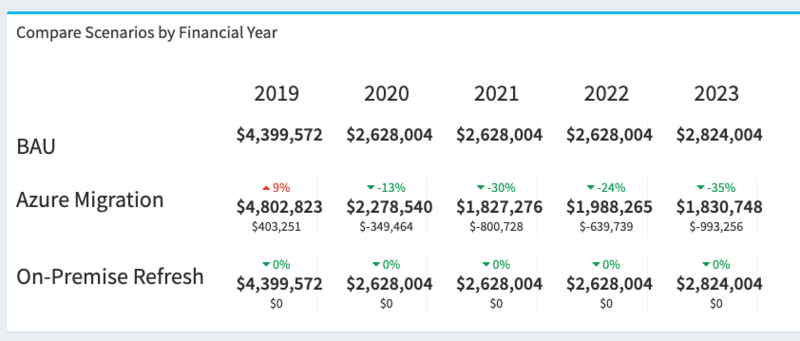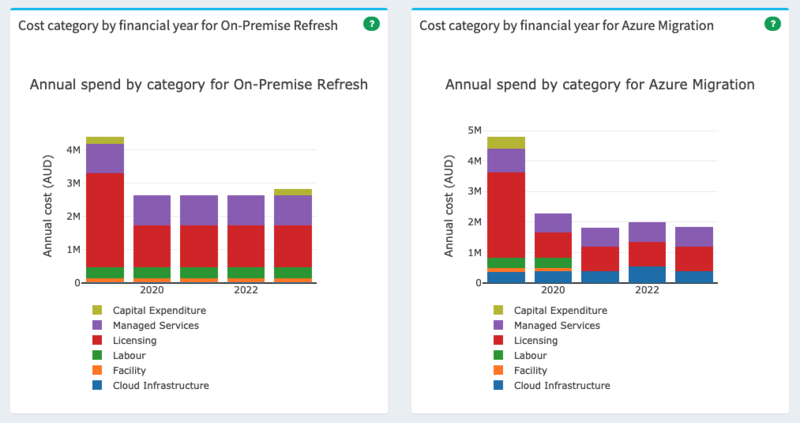Financial Overview

# Financial Overview Page

The Financial Overview is a roll up of the capital and operating costs per Financial Year for each Scenario.  They are compared against an imaginary BAU which is essentially all of the infrastructure that is not allocated to a scenario.

The BAU row shows the total spend in each financial year.  Each additional row represents a scenario and includes three numbers (top to bottom):

• Percentage Variance.  This is the percentage increase or decrease in this financial year when compared with the BAU scenario.
• Total Spend.  The total capital and operating expenditure in this financial year for the scenario.
• Nominal Variance.  The quantum of difference in that financial year when compared with BAU.Furthermore, the costs are visualised as a stacked column chart representing the total costs.  These let you explore how various categories of costs change over time.  During a cloud migration you'll see some costs reduce while others increase.  In general you can expect that during a period of transition there is an increase in cost followed by a commensurate overall reduction in costs after the transition.## Understanding the Percentage Increases

The financial overview illustrates any variance from business as usual (BAU) for the same financial year (FY). Under each Scenario a difference is identified. This is calculated as follows: Scenario - BAUDifference.

The percentage displayed is the percentage difference (either an increase or decrease) from the BAU figure and is calculated as follows: Difference / BAU * 100 = Percentage.

For example:

 Year 2020 BAU 44% (increase) \$1,256,169 \$553,417 Azure Migration \$1,809,586

Scenario (Azure Migration) 1809586 - BAU 1256169 =  Difference 553417

Difference 553417 / BAU 1256169 * 100 = Percentage 44%

In this example we identify an increase of 44% above the BAU expenditure for the year 2020 for the Azure Migration Scenario.

>